# Artifact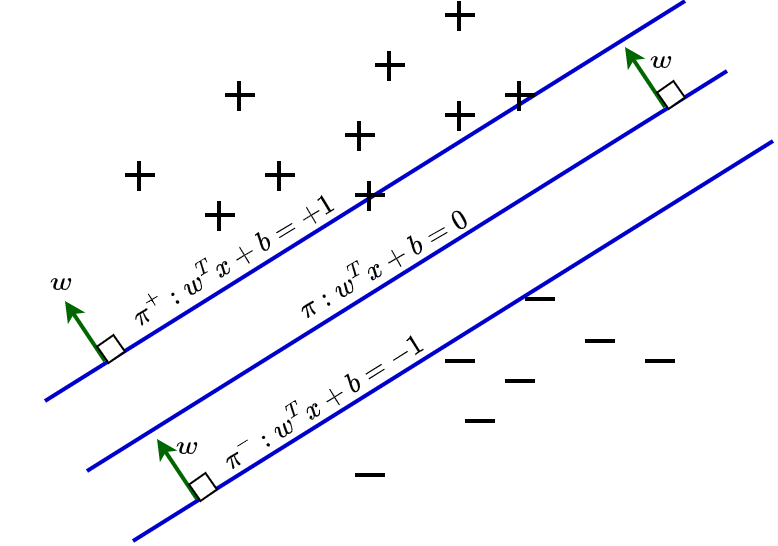## Support Vector Machines

What are Support Vector Machines? Support Vector Machines (SVM) is one of the most elegant methods used for classification and regression in machine learning (ML). We can use SVM when we have a bunch of objects/features which we want to classify. SVM performs the classification by drawing a hyperplane (Figure.1) to separate each category of …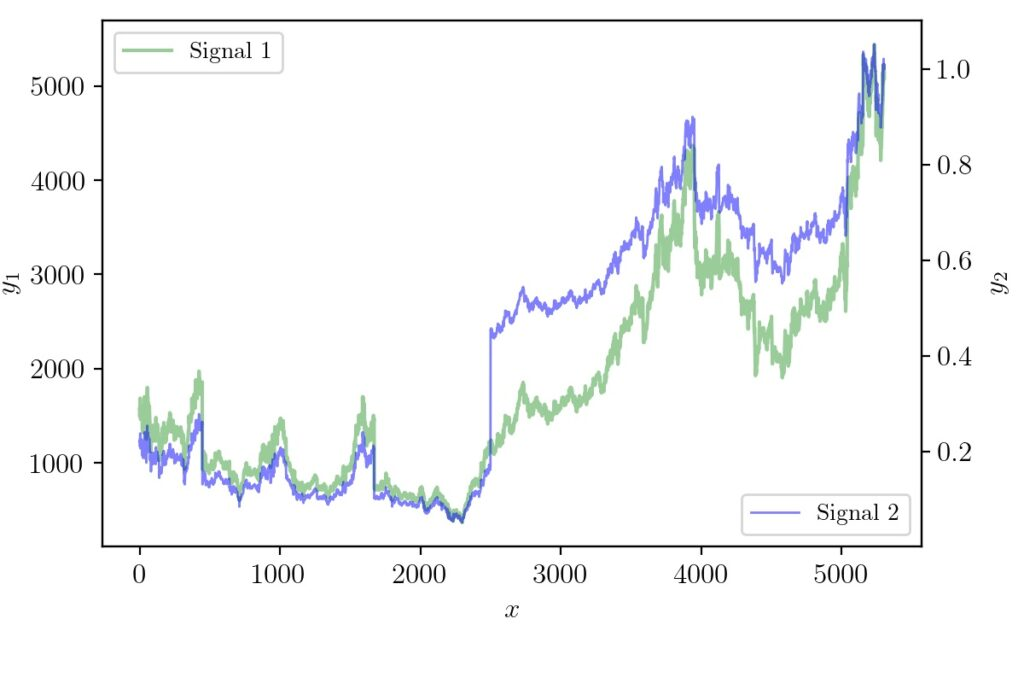## Python Matplotlib Tip: Overlapping Curves and Multiple Axes

In STEM fields it is often required to communicate ideas using graphs or infographics. Many times the dimensionality of data will be higher and require clever hacks to represent so that the reader gets the idea quickly. There have been systematic studies on how to do data visualization effectively. For data scientists or machine learning …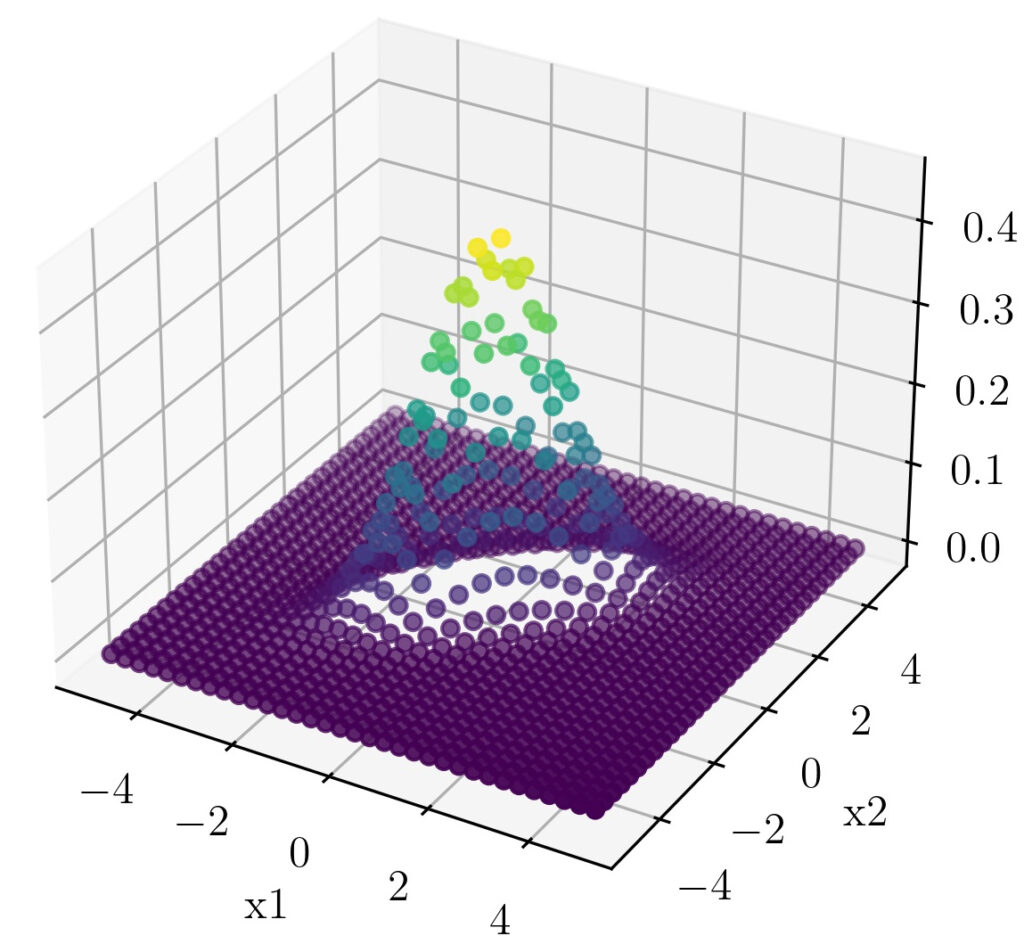## Hacking the Bivariate Gaussian Distribution

In one of our earlier posts, we have seen how we can visually relate the parts of the one-dimensional Gaussian distribution equation. In this post, we will follow the same strategy to understand the terms that comes up with a Multivariable Gaussian distribution. We will focus on the Bivariate Gaussian distribution as distributions of higher-order …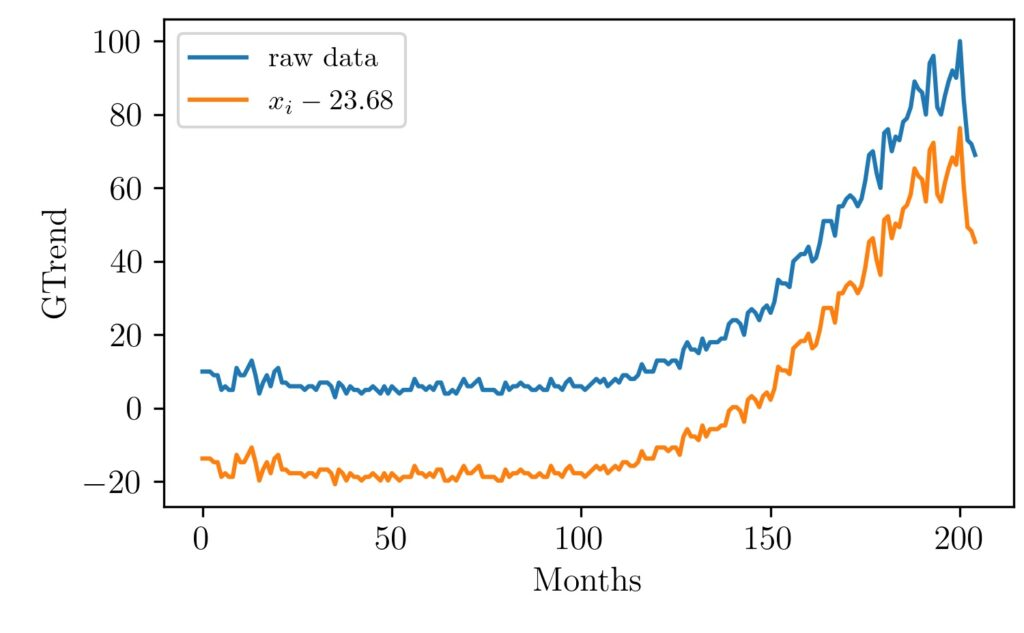## Visualizing MinMax Scaling

This article explains the minmax scaling operation using visual examples. Normalization of vectors, an array of values, signals is often used as a preprocessing step before many algorithms. For example, in machine learning, some types of algorithms are prone to different inherent scales of features. In such situations normalization is done to give the same …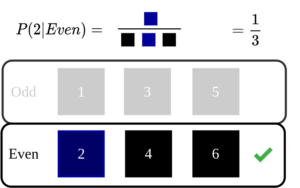## An Intuitive Explanation of Naive Bayes Classifier

Introduction In this post, let’s take a look at the intuition behind Naive Bayes Classifier used in machine learning. Naive Bayes classifier is one of the basic algorithms often encountered in machine learning applications. If linear regression was based on concepts from linear algebra and calculus, naive Bayes classifier mostly backed up by probability theory. …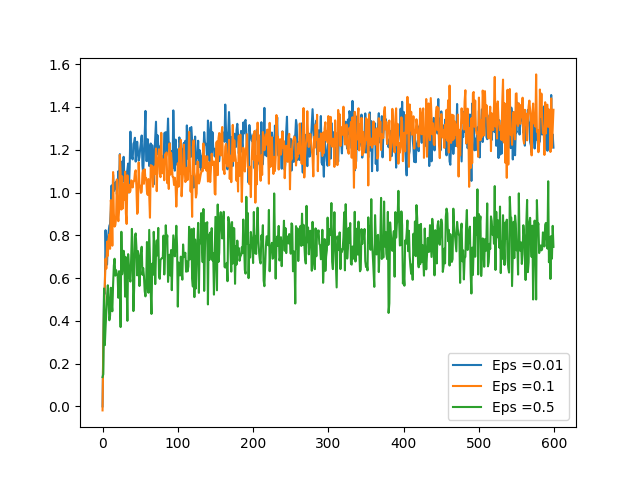## Epsilon Greedy Algorithm in Bandit Problems

Introduction Bandit problems are the simplest possible reinforcement learning scenario. Here the bandit machine can have k arms and pulling each arm leaves the user a reward. One of the arms will be giving higher rewards in the long run and moreover this pattern could be changing over a time period. Think of the scenario …